## Thursday, July 30, 2009

### Larry Zimmerman Plus

Larry Zimmerman is an extraordinary man, teacher, mind; one of those math teachers that infectiously inspires creativity and enthusiasm. As a teacher, he is alert, industrious, sensitive, clear, direct. He presents an entirely different model of teaching than anything I’ve seen before, and it made me wonder, if a little desperately, how my life would change if he had been my coach. He has recently retired from a long career at Brooklyn Tech and is pretty involved in the New York Math Circle (http://www.nymathcircle.org/)

My notes and quotes on him came from a workshop he taught on problem creation yesterday. My take on this part of his teaching is that it taps into students' (& teachers) metacognition (thinking about thinking, thinking about the fact that they are doing math while they're doing math), pattern seeking abilities and natural curiosity. I'm trying to approach planning by simply stating the questions I want to be prepared to ask during a lesson, as well as return to through the course of a unit. Here's an example of the list of questions that came from one initial problem (which I didn't initially even think was all that interesting.) I found the questions we came up with fascinating and pretty surprising.

“Theme”: How many distinct positive integer factors has the number 36?

“Variations” in no particular order:
- How many distinct positive integer factors has the number 37?
- List the factors of 36.
- What is the smallest positive integer which has the same number of factors as 36 (including 1 and itself)?
- How many distinct positive integer factors has the number 40?
- How many distinct positive integer factors has the number 49?
- How many distinct positive integer factors has the number 944?
- If x < y, and x divides y, can the number of factors of x be greater than or equal to the number of factors of y?
- Is there a positive integer for which the integer is less than the number of its factors?
- Can you find the number of factors of a number without enumerating them?
- Which positive integers have an even number of factors?
- Which positive integers have an odd number of factors?
- How many distinct positive integer factors of 36 are even/odd/perfect squares/multiples of 6/etc.?
Other inspired questions, not particularly related to the theme:
- Is zero an even integer?
- Is zero a factor of 5?
- Is 5 a factor of zero?
- Is 2 prime?
- What is the next even prime?
- Define a definition.
- What is the purpose of a definition?

## Wednesday, July 29, 2009

### Larry Zimmerman Quotes & Notes

“It is perhaps more important to be able to compose problems than to solve them.”
“Problem creation is the essence of mathematics.”
“The first effort is rarely if ever the final product.” (we must emphasize editing)
“Creating problems is theme and variations.”
“Determine what you are looking for and WRITE IT DOWN.”
“I don’t know what I want them to realize.”
“Beware of reinforced suspicion,” which is not a substitute for problem solving.
“If I’m 100% I will not forget it, I write it down.”
“There is nothing wrong with clarity by redundancy.”

Types of Problems
• Surprising
• Novel
• Fruitful
• Charming, imaginative, alluring
• Historically significant
• Haunting, musical
• Beautiful, elegant, sublime

Elements of Problems
1. a goal (construct, prove, maximize, minimize, classify, compare, compute)
2. given information
3. special rules (sometimes)

Some Big Ideas
- Plant a seed and then walk away until someone says something. Students and teachers struggle with silence, but it’s important and it saves time.
- Keep a notebook (both teachers and students) of interesting questions.
- We are well versed at turning a lot of words into symbols. Do the reverse.
- There are 3 varieties of equations: identities (always true), conditionals (sometimes true), and contradictions (never true)

### Sites of the Day

Arbitrary digits of pi in base 16
http://www.sciencenews.org/sn_arc98/2_28_98/mathland.htm

Online Encyclopedia of Integer Sequences: http://www.research.att.com/~njas/sequences/Seis.html

### Mary O'Keeffe Best Games Ever

Number Bracelets Game (adapted from Marilyn Burns at

Imagine that you have lots of beads, numbered from 0 through 9, as many as you want of each kind.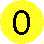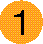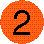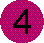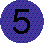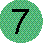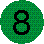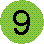Here are the rules for making a number bracelet:

• Pick a first and a second bead. They can have the same number.
• To get the third bead, add the numbers on the first and second beads. If the sum is more than 9, just use the last (ones) digit of the sum.
To get the next bead, add the numbers on the last two beads you used, and use only the ones digit. So to get the fourth bead, add the numbers on the second and third beads, and use the ones digit.
Keep going until you get back to the first and second beads, in that order.
• How long (or short) a bracelet can you make?
Other Questions:
How long is the longest?
How short is the shortest?
How many number bracelets are there?
- How many different starting points?
- How many different bracelets?
Does it necessarily repeat?
Extensions:
Change the mod/cutting number
Change the rule (from Fibonacci to some other sequence)
Why no bracelet of 6?
Why no bracelet of 5, 10, other factors of 60?
What if only use even numbers between 0 and 9?

Prime Number Monopoly
Materials: 2 dice, 5 cards (one for each of the first 5 prime #s) with the following costs and deed contracts:
Prime Number Deed:
Cost:
2 – \$250
3 – \$167
5 – \$100
7 – \$71
11 – \$45
The owner of this deed
splits the winnings
when another player gets a multiple of:
(prime number here)

Game: Roll two dice and can choose the amount of either number formed by those two digits. Can buy prime numbers. Then whenever another player chooses a number that is a multiple of your number, they split the \$ with you.

Goal: Get as much money as possible (there will be times when it will be to your advantage to choose the smaller number). Play for a set amount of time, clearly stated in advance.

Questions:
How did I work out the pricing?
What’s the best prime real estate buy?

### Mary O'Keeffe Quotes

“Math can and should be a social experience.”
“Get people comfortable with making mistakes and taking intellectual risks in public.”
“Give people powerfully compelling activities they will want to share with others, who will want to share them with others, etc.”
“Best way to learn is to share things with others.”
“I like to blurt out things when I’m not at all sure that I’m right.”
“We respect respect and we correct mistakes.”

### Mary O'Keeffe Book Recommendations

About Teaching Mathematics by Marilyn Burns
Aha! Gotcha by Martin Gardner
Aha! Insight by Martin Gardner
The Annotated Alice by Martin Gardner
Feynman and Asimov
Melisande by E. Nesbit
Mathematical People (interviews)
More Mathematical People (interviews)
Raymond Smullyan logic stories
Calculus By and For Young People by Don Cohen http://www.mathman.biz/

## Tuesday, July 28, 2009

### David Hankin Questions, many of them good & big ones

• What is geometry?
• When we study geometric figures, what are we concerned with?
• When we study geometric figures, what are we not concerned with?
• What kinds of motion (transformation) keep a figure “the same”?
• What is the maximum number of these motions (isometries) are necessary to get from one figure to another if they have the same orientation? If they have different orientation?
• What minds of motion change a figure?
• What is an angle?
• Given a diagram, what do you think might be true? (rather than given a diagram and some true things, prove this other thing)
• What is symmetry?
• Why do we study almost exclusively symmetric figures in geometry?
• Is a line segment symmetric?
• Is an angle symmetric?
• Are triangles symmetric?
• What can we say about the points on a perpendicular bisector?
• What happens when we find the perpendicular bisectors of the sides of a triangle?
• When is the circum center ON (/in/out) a triangle?
• What happens when we find the angle bisectors of a triangle?
• How many lines of symmetry does a quadrilateral have?
• Is it possible for a figure to have more than four lines of symmetry?
• How many lines of symmetry does a circle have?
• How do I draw a symmetry line through a circle?
• What happens when we draw the diameter through a point on a tangent line?
• What happens when we draw perpendicular chords through a diameter?
• What happens when we draw progressively smaller and smaller perpendicular chords?
• Problem: Given a triangle and side lengths, find the lengths of the segments formed by the incircles.
• Problem: Given a quadrilateral and side lengths, find the lengths of the segments formed by the incircles.
• Must a quadrilateral have a circumcircle?
• Reflection Problem: Given two points A & B, and a line (below them), what is the shortest path from point A to the line and then to B?
• Reflection Problem: In pool/minigolf, where do you aim so that A bounces once (twice, 3 times, 4 times back to A!) before hitting B? When are these possible/not?
• What has translational symmetry?
• Translation Problem: Two towns on opposite sides of a 1 mile wide river. Where should we put the bridge (to minimize the distance)? What if there are two nonparallel rivers?
• What letters are preserved under 180 rotation (/half turn/point reflection)?
• What geometric figures are preserved under point reflection? (which have point symmetry?)
• What geometric property follows from the fact that the letter Z has point symmetry?
• Point Reflection Problem: two circles intersect. Draw through the point of intersection a line which creates congruent chords in each circle.

### David Hankin Quotes

1. Majority rules in math right?
2. Did we prove it or did we just all agree?